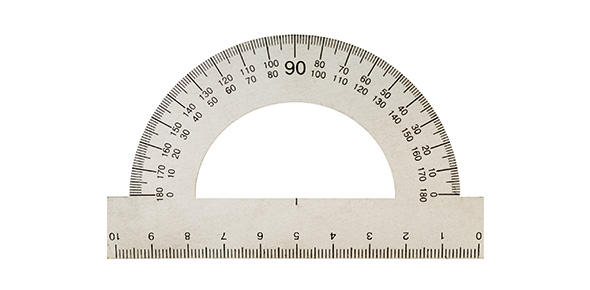# Measurement Quiz

14 Questions | Total Attempts: 754SettingsThis is a quiz that tests your knowledge about mass, weight, volume and density.

• 1.
Jose wants to figure out how much matter is in the key chain his dad gave him for his birthday. To figure this out what should Jose measure?
• A.

The mass of the key chain.

• B.

The weight of the key chain.

• C.

The volume of the key chain.

• D.

The density of the key chain.

• 2.
Why does a tennis ball weigh more on Earth than it does on the Moon?
• A.

Because the Moon has stronger gravity pulling down on the tennis ball.

• B.

Because the Earth has stronger gravity pulling down on the tennis ball.

• C.

Because the tennis ball has more mass on the Earth than on the Moon.

• D.

Because the tennis ball has more mass on the Moon than on the Earth.

• 3.
Amairany only has a little bit of space in her closet. She wants to figure out how many cube shaped boxes will fit inside. What should she measure.
• A.

The weight of the closet and the boxes.

• B.

The mass of the closet and the boxes.

• C.

The density of the closet and the boxes.

• D.

The volume of the closet and the boxes.

• 4.
What tool should you use to measure mass?
• A.

Triple Beam Balance

• B.

Spring Scale

• C.

• D.

Ruler

• 5.
When you are measuring the amount of matter in a certain amount of space you are measuring
• A.

Weight

• B.

Volume

• C.

Density

• D.

Mass

• 6.
What tool should you use to measure weight?
• A.

Triple Beam Balance

• B.

Spring Scale

• C.

• D.

Ruler

• 7.
What tool should you use to measure the volume of a cube?
• A.

Triple Beam Balance

• B.

Spring Scale

• C.

• D.

Ruler

• 8.
What tool should you use to measure the volume of a rock?
• A.

Triple Beam Balance

• B.

Spring Scale

• C.

• D.

Ruler

• 9.
If you measure an object and it is 10 grams what property did you measure?
• A.

Density

• B.

Mass

• C.

Volume

• D.

Weight

• 10.
If you measure an object and the measurement is 100 g/cm cubed what property did you measure?
• A.

Density

• B.

Mass

• C.

Volume

• D.

Weight

• 11.
If you measure an object and the measurement is 50 newtons what property did you measure?
• A.

Mass

• B.

Volume

• C.

Weight

• D.

Density

• 12.
If you measure an object and the measurement is 5 millimeters cubed what property did you measure?
• A.

Mass

• B.

Volume

• C.

Weight

• D.

Density

• 13.
Describe two types of errors that can happen when you are measuring the mass, weight or volume of an object.
• 14.
Give at least two ways that you can help avoid making errors when measuring an object's mass, weight or volume.
Related TopicsBack to top